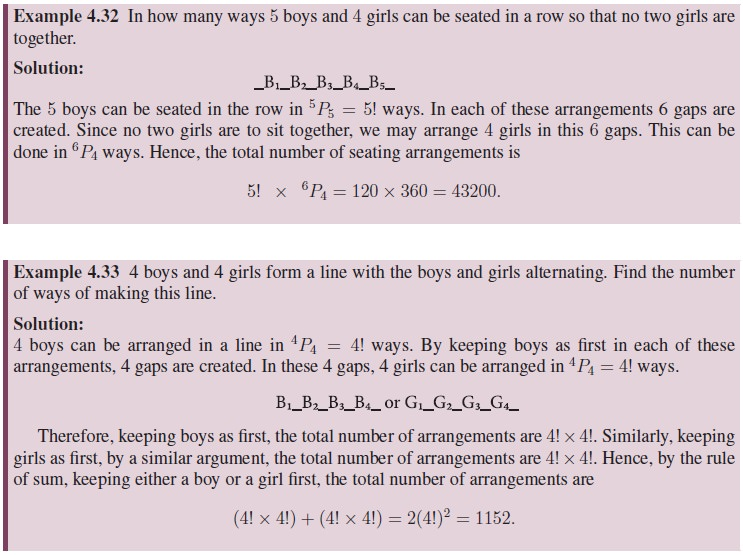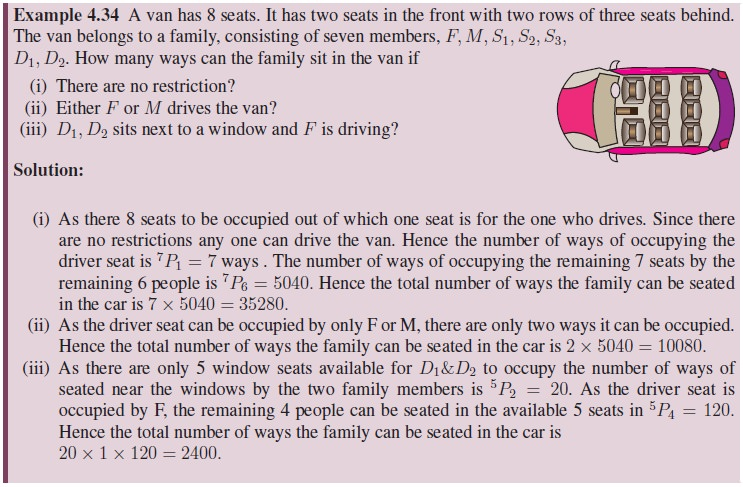Home | | Maths 11th std | Permutations

# Permutations

The number of permutations of 4 objects taken all at a time is 4 × 3 × 2 × 1 = 4! .

Permutations

What is a permutation ?

Permuations come in various disguises.

Suppose three friends A, B and C have to stand in line for a photograph. In how many order can they stand? Some of the possible arrangements (from left to right) are

A, B, C: A, C, B: B, A, C

B, C, A: C, B, A: C, A, B.Thus there are six possible ways in which they can arrange themselves for the photograph.

Thus if 3 objects have to be arranged in a row there are 3 × 2 × 1 = 3! possible permutations. The number of permutations of 4 objects taken all at a time is 4 × 3 × 2 × 1 = 4! Thus if n objects have to be arranged in a line there are n × (n 1) × (n 2) × · · · × 3 × 2 × 1 = n! possible arrangements or permutations.

Suppose you have 7 letters A,B,C,D,E,F and G. We want to make a 4 letter string. We have 7 choices for the 1st letter. Having chosen the first letter, we have 6 choices for the second letter. Proceeding this way, we have 4 choices for the 4th letter.

Hence, the number of permutations of 4 letters chosen from 7 letters is## 1. Permutations of distinct objects

In terms of function on any finite set say S = {x1, x2, ...xn}, a permutation can be defined as a bijective mapping on the set S onto itself. The number of permutation on the set S is the same as the total number of bijective mappings on the set S.

We denote the number of permutations by nPr.

Theorem 4.1: If n, r are positive integers and r n, then the number of permutations of n distinct objects taken r at a time is n (n 1) (n 2) · · · (n r + 1).

Proof. A permutation is an ordering. A permutation of n distinct objects taken r at a time is formed by filling of r positions, in a row with objects chosen from the given n distinct objects.There are n objects that can be filled in the first position. For the second position there are remaining n 1 objects. There are n 2 objects for the third position. Continuing like this until finally we place one of the (n (r 1)) possible objects in the rth position. By the rule of product we conclude nPr = n (n 1) (n 2) · · · (n r + 1).Theorem 4.3: The number of permutations of n different objects taken r at a time where repetition is allowed, is nr.We can fill the first position with n objects. For the second position (still we can use the object used in first position), there are n objects, and so on the rth position can be filled with n objects.By the rule of product, The number of permutations of n different objects taken r at a time when repetition allowed is n × n × n × · · · n (r times) = nr.

## 2. Properties of Permutations## 3. Objects always together (String method)

The number of permutations of n different objects, taken all at a time, when m specified objects are always together,

·        Consider a string of m specified objects as a single unit

·        Then we have (n m + 1) objects. Permute this (n m + 1) objects in (n m + 1)! ways.

·        Then permute the m specified objects between themselves in m! ways.

·        Finally, the answer is m! × (n m + 1)!.

## 4. No two things are together (Gap method)

To obtain the number of permutations of n different objects when no two of k given objects occur together and there are no restrictions on the remaining m = n k objects, we follow the procedure as follows:

·        First of all, arrange the m objects on which there is no restriction in a row. These m objects can be permuted in mPm = m! ways.

·        Then count the number of gaps between every two of m objects on which there is no restriction including the end positions. Number of such gaps will be one more than m that is (m + 1). In this m + 1 gaps, we can permute the k objects in m+1Pk ways.

·        Then the required number of ways are m! ×(m+1) Pk.To understand the next problem we now define, The Rank of a word in the dictionary.

It is the place at which the given word comes when writing all the strings formed by the letters of the given word in the dictionary order or lexicographic order.## 5. Permutations of not all distinct objects

Consider permuting the letters of the word JEE. In this case the letters of the word are not different. There are 2 E’s, which are of same kind. Let us treat, temporarily, the 2 E’s as different, say E1 and E2. The number of permutations of 3 different letters taken all at a time is 3!.Tags : Definition, Formula, Properties, Solved Example Problems, Exercise | Mathematics , 11th Mathematics : UNIT 4 : Combinatorics and Mathematical Induction
Study Material, Lecturing Notes, Assignment, Reference, Wiki description explanation, brief detail
11th Mathematics : UNIT 4 : Combinatorics and Mathematical Induction : Permutations | Definition, Formula, Properties, Solved Example Problems, Exercise | Mathematics